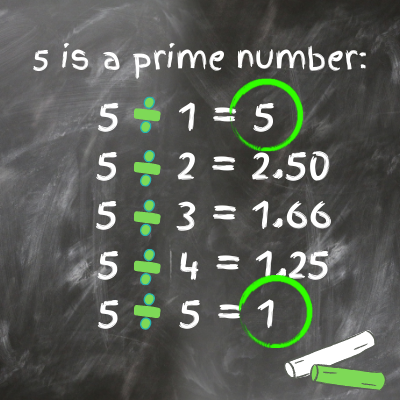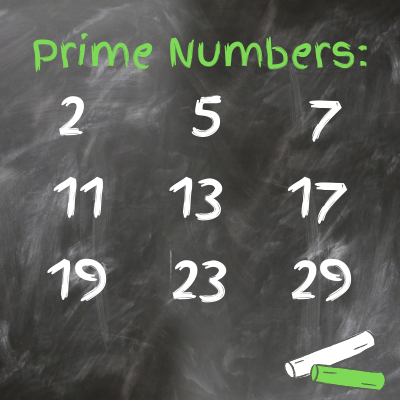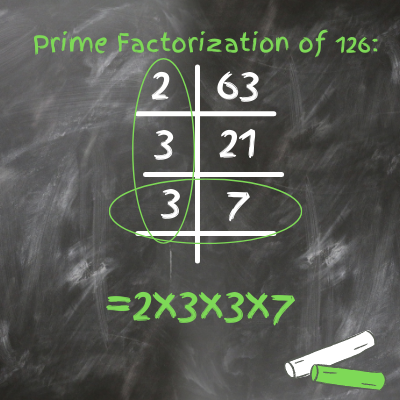# Prime Factorization

Lesa Steen, Benjamin Rosenthal
• Author
Lesa Steen
• Instructor
Benjamin Rosenthal
What is prime factorization? How about prime factors and a factor tree model? With this lesson you will learn the definition of prime factorization, explanation of prime factors, factor tree model, and how prime numbers are used. Updated: 06/28/2021

Show

## What is Prime Factorization?

Prime factorization can be used as both a noun and a verb. Essentially, it is the process of obtaining the unique prime numbers that multiply into a larger number. The resulting answer is also called the prime factorization. Though the prime factorization definition is simple, the process can look like a tricky topic on the surface; however, it can be broken down into manageable steps.

We have multiple methods for finding a number's prime factorization. Being able to quickly find a number's prime factorization helps with simplifying and combining fractions. Once we know the prime factorizations of two numbers, we can use that to easily find the greatest common factor and least common multiple of those numbers.An error occurred trying to load this video.

Try refreshing the page, or contact customer support.

Coming up next: How to Multiply and Divide Rational Expressions

### You're on a roll. Keep up the good work!

Replay
Your next lesson will play in 10 seconds
• 0:04 Prime Factorization
• 0:41 Factor Trees
• 4:21 Why Use Prime Factors?
• 5:06 Lesson Summary
Save Save

Want to watch this again later?

Timeline
Autoplay
Autoplay
Speed Speed

## Important Concepts in Prime Factorization:

Let's begin breaking down prime factorization into manageable steps by looking first at its components: prime numbers. Prime factors go hand in hand with prime numbers.

• A prime number is a number that can only be divided by 1 and itself evenly.
• Prime factors, explained in a little more detail, are prime numbers that can be multiplied to create a larger number.

5 is an example of a prime number because it can only be divided by 5 and 1 evenly.We also have a name for non-prime numbers:

• Composite numbers: a composite number is a positive whole number that is not prime and can be divided into smaller whole numbers.

Each composite number has a special series of prime numbers that can be multiplied to get the larger number. This is what makes the prime factors for a number special; the combination of prime factors is unique to each composite number.

To see what prime factorization means, we can apply it to a composite number. Let's start by going over the prime factors that make up 24.

We can check to see if we have the prime factors of a number by multiplying together that string of numbers. If the result is equal to our original number, then we have the factors. If the factors can only be divided by 1 and themselves then they are all prime factors.

For instance, if we look at the number 24:

• The prime factors would be 2 * 2 * 2 * 3.
• If we multiply that back, then we get 2 * 2 * 2 * 3 = 24.
• This combination of prime factors is unique to the number 24. We could not multiply the same prime factorization to get 26, for example. So, each prime factorization is unique to an individual number.

The prime numbers under 30 are very commonly used, so it may be helpful to memorize them.## Serial Factorization Method of Prime Factorization:

It is especially important to know common prime factors when working with the serial factorization method of prime factorization. With serial factorization, we factor out each prime number by beginning with the smallest prime number and increasing the size of the prime number until the remaining factor is prime.

Let's look at some prime factorization examples to help determine how to work with the serial factorization method.:

• If we have the number 96 and are looking for the prime factors that go into it we know we can first factor out a 2. It is the smallest prime factor that goes into 96, giving us 2 * 48.
• We can factor out another 2 from 48 as it is the smallest prime factor that goes into 48, giving us 2 * 2 * 24.
• Again, we can factor out a 2, leaving us with 2 * 2 * 2 * 12.
• We can factor out a 2 from 12 and are left with the following: 2 * 2 * 2 * 2 * 6.
• Finally, we can factor out a 2 from 6 and we are left with 2* 2 * 2 * 2 * 2 * 3.
• All the numbers in this number string are prime, so it is our prime factorization for the number 96.

As another example, here are the steps for finding the prime factorization of 126:

• 2 goes into 126, 63 times.
• 3 goes into 63, 21 times.
• 3 goes into 21, 7 times.
• Since 7 is a prime number, that is where we stop.
• The final answer is the prime factorization of 126 = 2 * 3 * 3 * 7.

We can also line prime factors up in a tee chart to help organize the process of serial factorization. We see that the prime factors are circled on the left side of the chart. The final number we end up with on the right side will also be a prime factor. Look at the example with the number 126.## Factor Tree Method of Prime Factorization:

The factor tree method is performed just as it sounds, by placing the factors in a tree shaped formation downward from the original number. The difference between the factor tree method and serial factorization is that instead of starting with the smallest prime factor that will go into the number, we can start with any combination that will go into the number. Once a prime factor is found, that branch of the tree comes to a stop.

Let's look at some prime factorization examples to help determine how to work with the factor tree model:Using the factor tree method with 96, we have the following steps:

• 96 can be broken down into 6 and 16.
• 6 can be broken down further into 2 * 3, which are both prime numbers. The branch of the tree stops on this side.
• The 16 can be broken down into 2 * 8. The 2 is prime so it stops.
• The 8 can be broken down into 2 * 4. The 2 is prime so it stops.
• The 4 can be broken down into 2 * 2.
• This leaves the final prime factorization for 96 as 2 * 2 * 2 * 2 * 2 * 3.

To unlock this lesson you must be a Study.com Member.

#### How do you find the prime factorization?

There are a couple methods for finding the prime factorization of a number. These include using a factor tree and serial factorization.

#### What is the definition for prime factorization?

Prime factorization (verb): It is the method in which we obtain the prime numbers that multiply into a larger number.

Prime factorization (noun): It is also what we call the final answer of one of these problems.

#### What is the prime factorization of 36?

The prime factorization of 36 is 2 * 2 * 3 * 3. You can get to this my breaking down 36 into 2 and 18. 2 is prime. 18 can then be broken down into 2 and 9. This 2 is your second 2 in the prime factorization. Then, the 9 can be broken down into 3 and 3. 3 is prime, leaving the final answer as 2 * 2 * 3 * 3

#### How do you find the prime factorization of a number?

You can find the prime factorization of a number by a few different methods: a factor tree or serial factorization. Using a factor tree, once a component can no longer break down evenly, it is a prime factor. For instance, finding the prime factorization of 12. First, break it into 3 * 4. 3 is prime, but 4 can be broken down further to 2 * 2. Since 2 is prime the prime factorization of 12 is 3 * 2* 2.

### Register to view this lesson

Are you a student or a teacher?